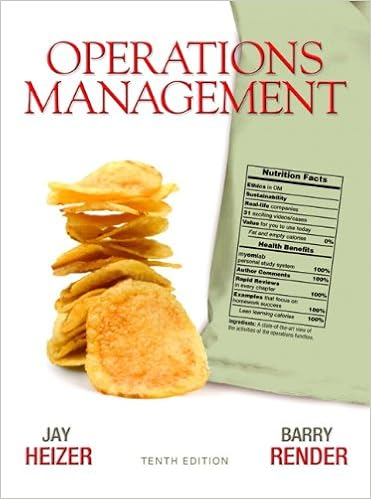Top Posters
Since Sunday
o
10
j
9
m
9
C
8
s
8
a
8
f
8
y
8
n
8
d
8
M
7

# What combination of x and y will yield the optimum for this problem?Minimize $3x +$15y, subject to ...

wrote...
Posts: 1447
Rep:
6 years ago
What combination of x and y will yield the optimum for this problem?
Minimize $3x +$15y, subject to (1) 2x + 4y ≤ 12 and (2) 5x + 2y ≤ 10 and (3) x, y ≥ 0.
A) x = 2, y = 0
B) x = 0, y = 0
C) x = 0, y = 3
D) x = 0, y = 5
E) x = 1, y = 5
Textbook## Operations ManagementEdition: 10thAuthors:
4 Replies
Replies
Answer verified by a subject expert
AlmeyricAlmeyric
wrote...
Top Poster
Posts: 929
Rep:
6 years ago

### Related Topics

wrote...
3 years ago
 thank you
Anonymous
wrote...
2 months ago
 Help! The answer is missing an explanation...
wrote...
2 months ago
 Since it's been a while since I answered, I have another question with its solution taken from my notes.SolutionWe know that the optimal solution occurs at corner points of the feasible region. Since this feasible region is bounded by ">=" constraints, we know that the 2 constraints will intersect with each other and with the x and y axes. We evaluate the objective function of each of these points (the intersection of the two constraints, the intersection of the constraints with the axes). We can graph the constraints to determine the coordinates of these points:We find that the corner points have the coordinates:1: (0, 5)2: (1, 2.5)3: (6, 0)Now that we have identified the coordinates, we evaluate each of the pair of coordinates with the objective function z = 3x + 15y:1: z = 3(0) + 15(5) = 0 + 75 = 752: z = 3(1) + 15(2.5) = 3 + 37.5 = 40.53: z = 3(6) + 15(0) = 18 + 0 = 18Thus, the combination of x and y that minimizes z is: (x, y) = (6, 0) and the objective function value is \$18.Did that help?otherwise try this video:
Explore
305 People Browsing
188 Signed Up Today
Related Images

176284257